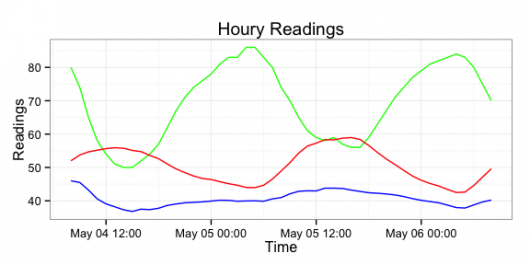Want to share your content on R-bloggers? click here if you have a blog, or here if you don't.

I’ve bumped up the version number of `Rforecastio` (github) to `1.1.0`. The new features are:

• removing the SSL certificate bypass check (it doesn’t need it anymore)
• using `plyr` for easier conversion of JSON->data frame
• adding in a new `daily` forecast data frame
• roxygen2 inline documentation
```library(Rforecastio) library(ggplot2) library(plyr)   # NEVER put API keys in revision control systems or source code! fio.api.key= readLines("~/.forecast.io")   my.latitude = "43.2673" my.longitude = "-70.8618"   fio.list <- fio.forecast(fio.api.key, my.latitude, my.longitude)   fio.gg <- ggplot(data=fio.list\$hourly.df, aes(x=time, y=temperature)) fio.gg <- fio.gg + labs(y="Readings", x="Time", title="Houry Readings") fio.gg <- fio.gg + geom_line(aes(y=humidity*100), color="green") fio.gg <- fio.gg + geom_line(aes(y=temperature), color="red") fio.gg <- fio.gg + geom_line(aes(y=dewPoint), color="blue") fio.gg <- fio.gg + theme_bw() fio.gg``````fio.gg <- ggplot(data=fio.list\$daily.df, aes(x=time, y=temperature)) fio.gg <- fio.gg + labs(y="Readings", x="Time", title="Daily Readings") fio.gg <- fio.gg + geom_line(aes(y=humidity*100), color="green") fio.gg <- fio.gg + geom_line(aes(y=temperatureMax), color="red") fio.gg <- fio.gg + geom_line(aes(y=temperatureMin), color="red", linetype=2) fio.gg <- fio.gg + geom_line(aes(y=dewPoint), color="blue") fio.gg <- fio.gg + theme_bw() fio.gg```